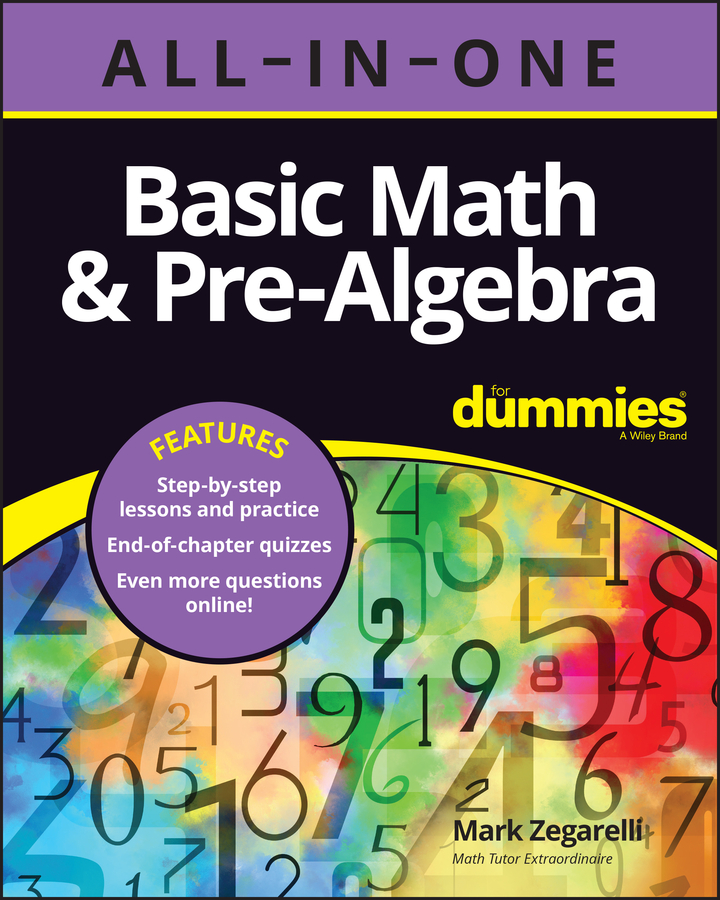##### Basic Math & Pre-Algebra All-in-One For Dummies (+ Chapter Quizzes Online)In math, parentheses — ( ) — are often used to group together parts of an expression. This helps you to find the order of precedence when you work with equations. When it comes to evaluating expressions that contain parentheses, you can follow these steps:

1. Evaluate the contents of parentheses, from the inside out.

2. Evaluate the rest of the expression.

## Big Four expressions with parentheses

Similarly, suppose you want to evaluate (1 + 15 ÷ 5) + (3 – 6) · 5. This expression contains two sets of parentheses, so evaluate these from left to right. Notice that the first set of parentheses contains a mixed-operator expression, so evaluate this in two steps starting with the division:

= (1 + 3) + (3 – 6) · 5

= 4 + (3 – 6) · 5

Now evaluate the contents of the second set of parentheses:

= 4 + –3 · 5

Now you have a mixed-operator expression, so evaluate the multiplication (–3 · 5) first:

= 4 + –15

Finally, evaluate the addition:

= –11

So (1 + 15 ÷ 5) + (3 – 6) · 5 = –11.

## Expressions with exponents and parentheses

As another example, try this out:

1 + (3 – 62 ÷ 9) · 22

Start out by working only with what’s inside the parentheses. The first thing to evaluate there is the exponent, 62:

= 1 + (3 – 36 ÷ 9) · 22

Continue working inside the parentheses by evaluating the division 36 ÷ 9:

= 1 + (3 – 4) · 22

Now you can get rid of the parentheses altogether:

= 1 + –1 · 22

At this point, what’s left is an expression with an exponent. This expression takes three steps, starting with the exponent:

= 1 + –1 · 4

= 1 + –4

= –3

So 1 + (3 – 62 ÷ 9) · 22 = –3.

## Expressions with parentheses raised to an exponent

Sometimes, the entire contents of a set of parentheses are raised to an exponent. In this case, evaluate the contents of the parentheses before evaluating the exponent, as usual. Here’s an example:

(7 – 5)3

First, evaluate 7 – 5:

= 23

With the parentheses removed, you’re ready to evaluate the exponent:

= 8

Once in a rare while, the exponent itself contains parentheses. As always, evaluate what’s in the parentheses first. For example,

21(19 + 3 –6)

This time, the smaller expression inside the parentheses is a mixed-operator expression. The part that you need to evaluate first is underlined:

= 21(19 + –18)

Now you can finish off what’s inside the parentheses:

= 211

At this point, all that’s left is a very simple exponent:

= 21

So 21(19 + 3 –6) = 21.

Technically, you don’t need to put parentheses around the exponent. If you see an expression in the exponent, treat it as though it had parentheses around it. In other words, 2119 + 3 –6 means the same thing as 21(19 + 3 –6).

## Expressions with nested parentheses

Occasionally, an expression has nested parentheses: one or more sets of parentheses inside another set. Here is the rule for handling nested parentheses:

When evaluating an expression with nested parentheses, evaluate what’s inside the innermost set of parentheses first and work your way toward the outermost parentheses.

For example, suppose you want to evaluate the following expression:

2 + (9 – (7 – 3))

The contents of the innermost set of parentheses are underlined, so evaluate these contents first:

= 2 + (9 – 4)

Next, evaluate what’s inside the remaining set of parentheses:

= 2 + 5

Now you can finish things off easily:

= 7

So 2 + (9 – (7 – 3)) = 7

As a final example, here’s an expression that requires everything from this chapter:

4 + (–7 · (2(5 – 1) – 4 · 6))

This expression is about as complicated as you’re ever likely to see in pre-algebra: one set of parentheses containing another set, which contains a third set. To start off, evaluate the underlined part of the equation, which is in the third set of parentheses:

= 4 + (–7 · (24 – 4 · 6))

Now, what’s left is one set of parentheses inside another set. Again, work from the inside out. The smaller expression here is 24 – 4 · 6, so evaluate the exponent first, then the multiplication, and finally the subtraction:

= 4 + (–7 · (16 – 4 · 6))

= 4 + (–7 · (16 – 24))

= 4 + (–7 · –8)

Only one more set of parentheses to go:

= 4 + 56

At this point, finishing up is easy:

= 60

Therefore, 4 + (–7 · (2(5 – 1) – 4 · 6)) = 60.